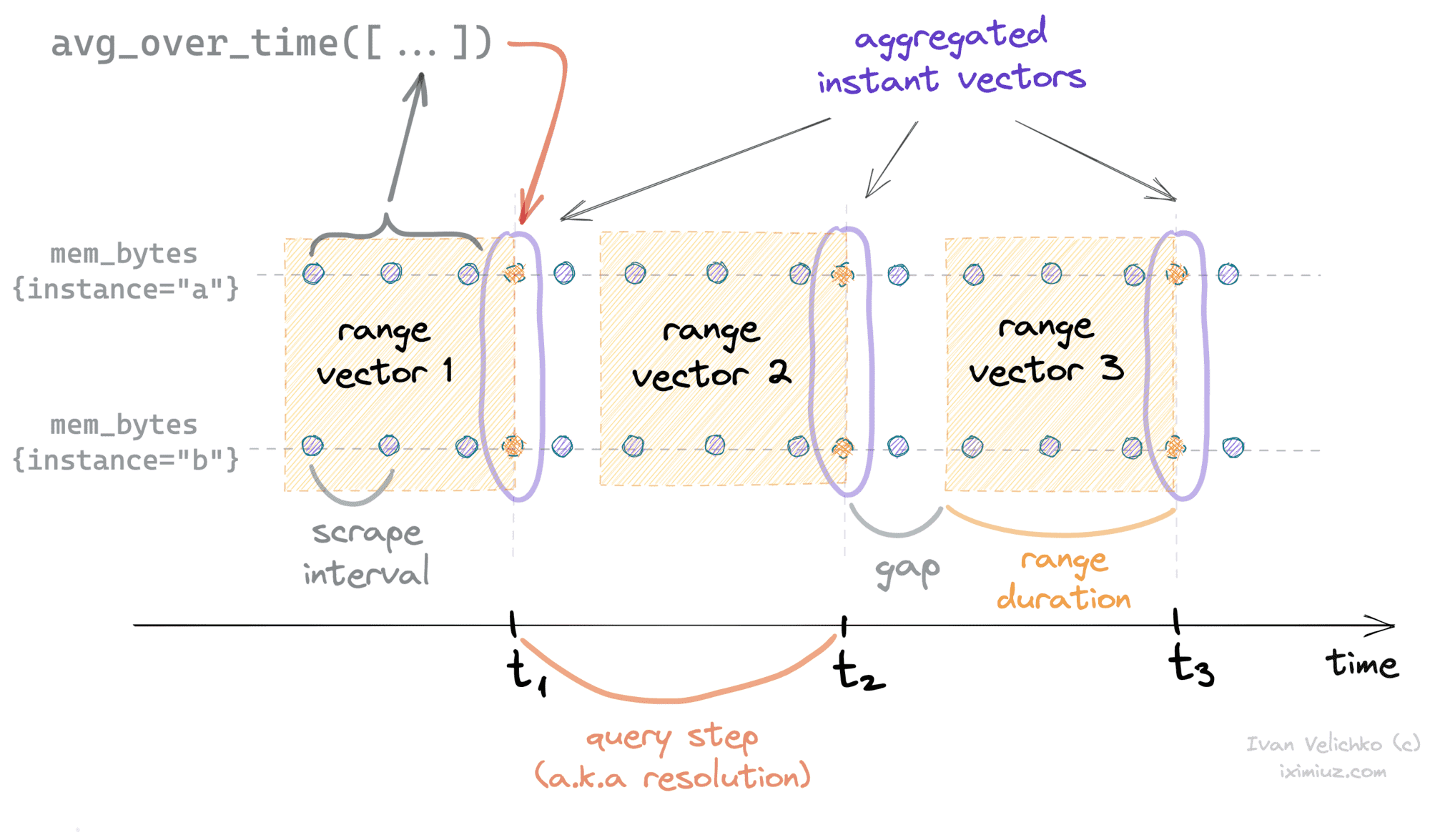When you have a long series of numbers, such as server memory consumption scraped 10-secondly, it's a natural desire to derive another, probably more meaningful series from it, by applying a moving window function. For instance, moving average or moving quantile can give you much more readable results by smoothing some spikes.

Prometheus has a bunch of functions called `<smth>_over_time()`. They can be applied only to range vectors. It essentially makes them window aggregation functions. Every such function takes in a range vector and produces an instant vector with elements being per-series aggregations.

For people like me who normally grasp code faster than text, here is some pseudocode of the aggregation logic:

``````# Input vector example.
range_vector = [
({"lab1": "val1", "lab2": "val2"}, [(12, 1624722138), (11, 1624722148), (17, 1624722158)]),
({"lab1": "val1", "lab2": "val2"}, [(14, 1624722138), (10, 1624722148), (13, 1624722158)]),
({"lab1": "val1", "lab2": "val2"}, [(16, 1624722138), (12, 1624722148), (15, 1624722158)]),
({"lab1": "val1", "lab2": "val2"}, [(12, 1624722138), (17, 1624722148), (18, 1624722158)]),
]

# agg_func examples: `sum`, `min`, `max`, `avg`, `last`, etc.

def agg_over_time(range_vector, agg_func, timestamp):
# The future instant vector.
instant_vector = {"timestamp": timestamp, "elements": []}

for (labels, samples) in range_vector:
# Every instant vector element is
# an aggregation of multiple samples.
sample = agg_func(samples)
instant_vector["elements"].append((labels, sample))

# Notice, that the timestamp of the resulting instant vector
# is the timestamp of the query execution. I.e., it may not
# match any of the timestamps in the input range vector.
return instant_vector
``````

Almost all the functions in the aggregation family accept just a single parameter - a range vector. It means that the over time part, i.e., the duration of the aggregation period, comes from the range vector definition itself.

The only way to construct a range vector in PromQL is by appending a bracketed duration to a vector selector. E.g. `http_requests_total[5m]`. Therefore, an `<agg>_over_time()` function can be applied only to a vector selector, meaning the aggregation will always be done using raw scrapes.

Another motivation for aggregating metrics over time comes from the fact that instant vectors of raw scrapes often are just glimpses of the actual state of your system. To facilitate graph plotting, Prometheus offers range API queries. Logically, a response of a range API query consists of a series of instant vectors, where each vector is one resolution step away from another. Depending on how lucky you are, you can miss important insights hidden in between any two consecutive vectors.

Unlike scalar elements of an instant vector, each element of the range vector is actually an array. Elements of such an array are scrape values falling into the corresponding time bucket. By applying an `<agg>_over_time` function to these buckets, we achieve a moving window effect that potentially can incorporate all data points without leaving any gaps. If the window duration is at least one resolution step long, none of the available data points will be discarded.Grafana tip: use `\$__interval` built-in variable for your range vector durations.

### min, max, avg, sum, stddev, stdvar over time

The naming makes the purpose of these functions quite obvious. For instance, `avg_over_time()` is what you may use to compute a moving average of some metric. Similarly, `stddev_over_time()` can be used to produce a moving standard deviation.

However, there is always a caveat. Prometheus supports different logical types of metrics - gauges, counters, histograms, and summaries. Surprisingly or not, physically, there is no difference between these types. All of them are just stored as series of float numbers. PromQL doesn't really distinguish between the logical types of metrics either. What only matters for PromQL is an expression type. I.e., it wouldn't allow you to call a function that expects an instant vector with a range vector argument. However, a range vector of gauges is physically indistinguishable from a range vector of counters. And here we go...

functions `min_over_time()`, `max_over_time()`, `avg_over_time()`, `sum_over_time()`, `stddev_over_time()`, and `stdvar_over_time()` makes sense to use only with gauge metrics.

Just think of it for a second. If you call, say, `min_over_time()` on a range vector of counters, it'd always return the left-most sample.

### quantile_over_time()

`quantile_over_time(scalar, range-vector)` - this is the only one that takes two parameters: a scalar defining the quantile to compute and a range vector.

### last_over_time()

`last_over_time(http_requests_total[5m])` is pretty much the same as just `http_requests_total`. If we take the very last sample from every element of a range vector, the resulting vector will be identical to a regular instant vector query. The only usage that comes to mind is to be able to manipulate the lookback window on the fly.

While constructing an instant vector, Prometheus looks up to 5 minutes back from the queried timestamp. For every metric, the very last sample falling into the `[t - 5m, t]` time range is then added to the resulting vector. The `5m` delta is a heuristical value that can be changed by providing `--query.lookback-delta` command-line parameter. However, changing it would require a server restart. Via using `last_over_time(<vector_selector>[<duration>])` we can control the lookback delta duration at runtime.

### count_over_time()

`count_over_time()` counts the number of samples for each element of the range vector. The actual values of the samples are disregarded. Thus, the resulting instant vector will just represent the number of scrapes per series falling into the requested time frame.